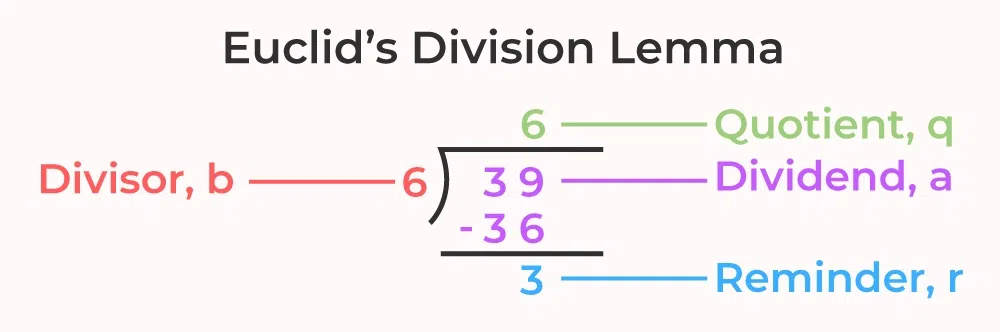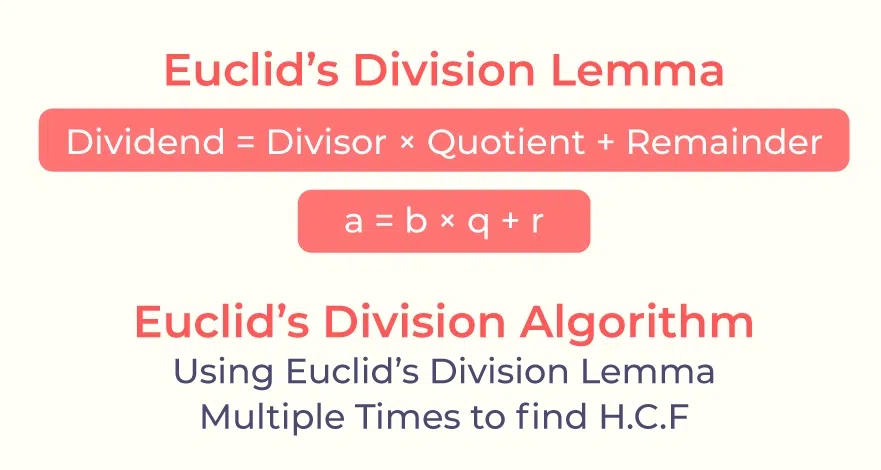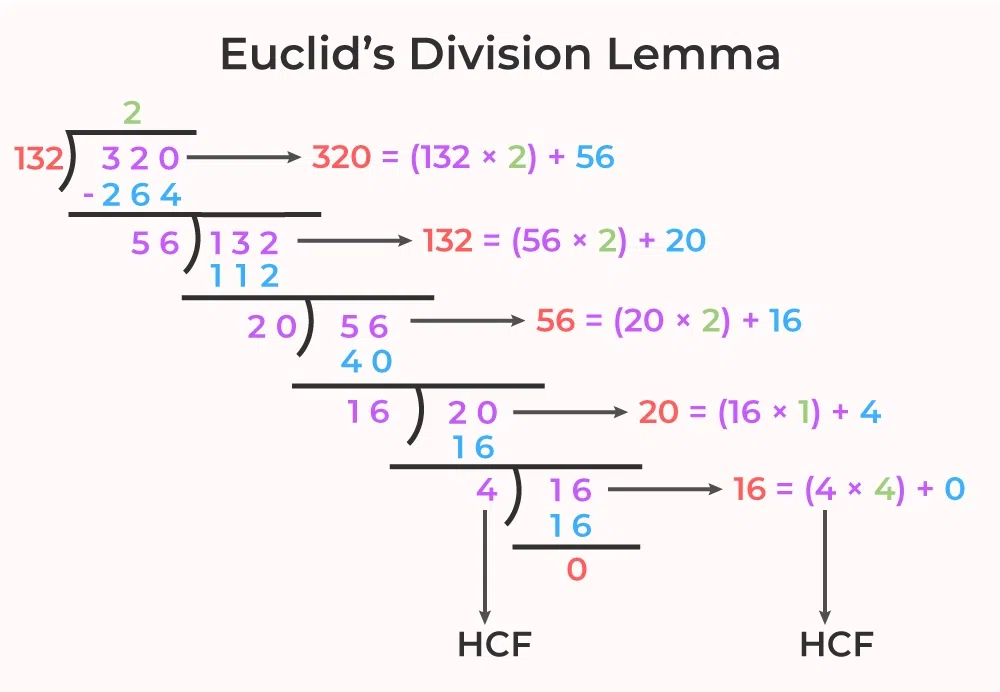GeeksforGeeks App
Open AppBrowser
Continue

# Euclid’s Division Lemma

Euclid’s Division Lemma is one of the fundamental theorems proposed by the ancient Greek mathematician Euclid. This theorem explains that for any two integers a and b, we have other two positive integers q and r such that, a = bq + r. With the help of Euclid’s Division Lemma an algorithm is defined i.e., Euclid’s Division Algorithm which is used to find the HCF of any two numbers. In this algorithm, apply Euclid’s Division Lemma multiple times to get the HCF of two numbers.

To understand Euclid’s Division Algorithm we first need to understand Euclid’s Division Lemma. Lemma is like a theorem and we will learn about Euclid’s Division Lemma, Euclid’s Division Algorithm, and others in detail in this article.

## Euclid’s Division Lemma Definition

Euclid’s Division Lemma gives the relation between the various components of Division. It explains that for any two positive integers say a and b there exist two unique integers q and r such that a = bq + r. In this method, we call q the quotient of the division, and r is the remainder of the division.

We have learned the division algorithm as,

Dividend = Divisor × Quotient + Remainder

This is nothing but another name for Euclid’s Division Lemma

Now let’s look at the statement of Euclid’s Division Lemma

### Statement of Euclid’s Division Lemma

For any two positive integers a and b, there exists a unique set of integers q and r, such that:

a = b q + r

Where,

• q is called the quotient, and
• r is called the remainder, 0 ≤ r < b.

Let’s consider an example of Euclid’s Division Lemma for better understanding.

Here, the given numbers are, 39(=a) and 6(=b) we can write it in a = bq + r form as,

39 = 6×6 + 3

where, quotient(q) is 6 and remainder(r) is 3.Now, the Euclid Division Lemma is, a = b × (q + r) can be written as,

Dividend = (Divisor × Quotient) + Remainder

• Euclid’s Division Lemma has many Applications related to the Divisibility of Integers
• It can be used to find the HCF of two numbers.

## Proof of Euclid’s Division Lemma

For any two positive integers a and b, there exist unique integers q and r such that a = bq + r, where 0 ≤ r < b.”

To prove Euclid’s Division Lemma, we need to establish two main parts: the existence and uniqueness of the integers q and r.

### Existence

We will prove the existence of integers q and r such that a = bq + r, where 0 ≤ r < b.

Consider the set S = {a – bk | k is an integer and a – bk ≥ 0}. S represents the set of all possible remainders when a is divided by b.

If S is empty, then there are no possible remainders greater than or equal to 0. However, this contradicts the fact that itself is an element of S (since a – b × 0 = a), and therefore, S cannot be empty.

Now, let’s assume that S contains a positive integer. In other words, there exists some k such that a – bk = r > 0. We can rewrite this equation as a = bq + r, where q = k and r is the remainder.

The only remaining case is when S contains negative integers only. Suppose a – bx < 0 for all integers x. This implies that a < bx for all x, which further implies a < b × 0. But this contradicts the fact that a is a positive integer. Hence, it is not possible for S to contain negative integers only.

Therefore, there must be a non-negative integer r in S. Hence, we have proven the existence part of Euclid’s Division Lemma.

### Uniqueness

We will now prove the uniqueness of the integers q and r in Euclid’s Division Lemma.

Suppose there exist two pairs of integers (q₁, r₁) and (q₂, r₂) such that a = bq₁ + r₁ and a = bq₂ + r₂, where 0 ≤ r₁ < b and 0 ≤ r₂ < b.

Subtracting these two equations, we get:

bq₁ + r₁ – (bq₂ + r₂) = 0

Simplifying, we have:

b(q₁ – q₂) + (r₁ – r₂) = 0

Now, since b(q₁ – q₂) is divisible by b, the left-hand side of the equation is divisible by b. Therefore, (r₁ – r₂) must also be divisible by b.

But we know that 0 ≤ r₁ < b and 0 ≤ r₂ < b, which means the absolute difference between r₁ and r₂ is less than b. Therefore, the only way (r₁ – r₂) can be divisible by b is if r₁ = r₂.

Hence, we conclude that r₁ = r₂. Substituting this back into the original equations, we get:

a = bq₁ + r₁ = bq₂ + r₁

This implies bq₁ = bq₂, and since b is a positive integer, we can cancel it from both sides to get q₁ = q₂.

Therefore, we have shown that if (q₁, r₁) and (q₂, r₂) are two pairs of integers satisfying a = bq₁ + r₁ and a = bq₂ + r₂, then q₁ = q₂ and r₁ = r₂. This establishes the uniqueness part of Euclid’s Division Lemma.

Hence, we have proven both the existence and uniqueness of the integers q and r in Euclid’s Division Lemma.

## What is Euclid’s Division Algorithm?

The process of finding the HCF of two numbers using Euclid’s Division Lemma is called “Euclid’s Division Algorithm”. In this process, Euclid’s Division Lemma is used in succession several times to find the HCF of the two numbers.

The image added below states Euclid’s Division Algorithm.Euclid’s Division Algorithm is one of the most common division algorithms taught to students to divide any number. An algorithm is a set of instructions provided to perform a specific task it repeats certain steps to complete a task. While applying Euclid’s Division Lemma we came across the condition that, for two integers a and b (a<b), there exists two integers q and r such that,

a = bq + r

After studying the above relation we can say that any number k which is the common factor of a and b is the common factor of r also. We use this concept to find the HCF of two numbers.

### Euclid’s Division Algorithm Example: Finding HCF

We can easily find the HCF of two numbers 135 and 275 by applying Euclid’s Division Lemma on these two numbers multiple times i.e. we can directly say that we can find the HCF of 135 and 275 by applying Euclid’s Division Algorithm. Follow the following steps to find the HCF.

Step 1: Use Euclid’s Division Lemma on 135 and 275

275 = 135(2) + 5

As we know that HCF is the highest factor of 135 and 275 and also any factor of 275 and 135 is also a factor of 5. So further applying Euclid’s Division Lemma on 135 and 5. (This process is repeated until the remainder r in Euclid’s Division Lemma becomes zero(0) and the remainder in the second last step is the required HCF of given two numbers.

Step 2: Repeating Euclid’s Division Lemma further

135 = 5(27) + 0

As now the remainder is zero we can say that the HCF of 135 and 275 is 5

## Generalizing Euclid’s Division Algorithm

Now we can generalize Euclid’s Division Algorithm for finding the HCF of any number. Assume that we have to find the HCF of two random numbers a and b then their HCF is found by applying Euclid’s Division Lemma multiple times until the remainder obtained is zero (0). This can be understood as,

a = b(q1) + r1

b = r1(q2) + r2

r1 = r2(q3) + r3

rn−2 = rn−1(qn) + rn

rn−1 = rn(qn+1) + 0

Now the HCF of a and b is the remainder obtained in the second last step, i.e., the HCF (a, b) = rn

## Shortcut Method for Euclid’s Division Algorithm

We also have a shortcut method of finding the HCF of two numbers this method uses the concept of division to find the HCF. We know that,

Dividend = Divisor × Quotient + Remainder

Now we divide the given numbers (say and b) accordingly the bigger number with the smaller number and we obtained a quotient and a remainder. Now this remainder becomes the divisor and the previous divisor becomes the dividend and further, the division process is carried out.

This process is repeated till the remainder of the division is zero. And the quotient when the remainder becomes zero is the HCF of the two numbers a and b.

The following illustration shows the calculation of HCF of 132 and 320 using the shortcut method.• Thus, the HCF of 132 and 320 is 4.

## Solved Examples on Euclid’s Division Lemma

Example 1: Find the quotient and remainder when 73 is divided by 9 using Euclid’s Division Algorithm.

Solution:

Given: Dividend = 73, Divisor = 9

Using Euclid’s Division Lemma, Divide 73 by 9

⇒ 73 = 9 × 8 + 1

Therefore, when 73 is divided by 9, the quotient is 8 and the remainder is 1.

Example 2: Find the quotient and remainder when 315 is divided by 17 using Euclid’s Division Algorithm.

Solution:

Given: Dividend = 315, Divisor = 17

Using Euclid’s Division Lemma, Divide 315 by 17

⇒ 315 = 17 × 18 + 9

Thus, quotient is 18 and remainder is 9.

Example 3: Use Euclid’s Division Algorithm to Find the HCF of 867 and 255.

Solution:

Step 1: Since 867 > 255, we apply the Division Lemma on 867 and 255,

867 = 255 × 3 + 102

Step 2: Since the Remainder 102 ≠ 0, we apply the division lemma further on 255 and 102

255 = 102 × 2 + 51

This step is repeated until the remainder becomes zero.

Step 3: Since the Remainder 51 ≠ 0, we apply the division lemma further on 102 and 51

102 = 51 × 2 + 0

Now the remainder is zero, so our process stops. Since the divisor at this stage is 51, the HCF of 255 and 867 is 51.

Example 4: Use Euclid’s Division Algorithm to Find the HCF of 4052 and 12576.

Solution:

Step 1: Since 12576 > 4052, we apply the Division Lemma on 867 and 255,

12576 = 4052 × 3 + 420

Step 2: Since the Remainder 420 ≠ 0, we apply the division lemma to 4052 and 420,

4052 = 420 × 9 + 272

Step 3: Since the Remainder 272 ≠ 0, we apply the division lemma to 420 and 272

420 = 272 × 1 + 148

This step is repeated until the remainder becomes zero.

420 = 272 × 1 + 148

Since the Remainder 148 ≠ 0, we apply the division lemma to 272 and 148.

272 = 148 × 1 + 124

Since the Remainder 124 ≠ 0, we apply the division lemma to 148 and 124.

148 = 124 × 1 + 24

Since the Remainder 24 ≠ 0, we apply the division lemma to 124 and 24.

124 = 24 × 5 + 4

Since the Remainder 4 ≠ 0, we apply the division lemma to 24 and 4.

24 = 4 × 6 + 0

Now the remainder becomes zero, so our process stops. Since the divisor at this stage is 4, the HCF of 4052 and 12576 is 4.

## FAQs on Euclid’s Division Algorithm

### Q1: What is Euclid’s Division Lemma?

Euclid’s Division Lemma is the basic theorem of mathematics that says for any two positive integers a and b, we have two integers q and r such that,

a = bq + r

### Q2: What is Euclid’s Division Algorithm?

Euclid’s Division Algorithm is an algorithm which is used to find the HCF of two numbers by using Euclid’s Division Lemma in succession till the remainder(r) is made zero.

### Q3: What is the difference between Euclid’s Division Lemma and  Euclid’s Division Algorithm?

Euclid’s Division Lemma is the basic theorem of Euclid’s Division whereas Euclid’s Division Algorithm is an Algorithm which uses Euclid’s Division Lemma to find the HCF of two numbers.

### Q4: What is the use of Euclid’s Division Algorithm?

Euclid’s Division Algorithm is used to find the HCF of two or more numbers.

### Q5: What is the formula of the Division Algorithm?

The formula of the Division Algorithm is,

Dividend = Divisor × Quotient + Remainder